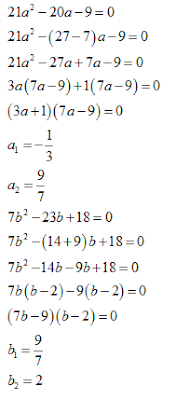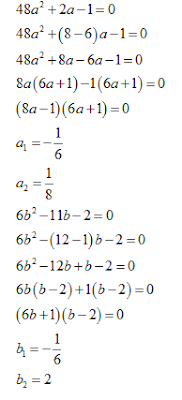# Quadratic Equations for SBI PO 2017: Part-3

1. In the following question two equations (A) and (B) given. You have to solve both:
a. 21a² - 20a - 9 = 0
b. 7b² - 23b + 18 = 0

i.  If a > b
ii. If a < b
iii.If a = b or C.N.E
iv. If a > b
v.  If a < b

2. In the following question two equations (A) and (B) given. You have to solve both:
a. 48a² + 2a -1 = 0
b. 6b² - 11b - 2 = 0

i.  If a < b
ii. If a > b
iii.If a > b
iv. If a < b
v.  If a = b or C.N.E

3. In the following question two equations (A) and (B) given. You have to solve both:
a. 9a² - 24a + 143 = 0
b. 9b² + 50b - 91=0

i.  If a > b
ii. If a < b
iii. If a > b
iv. If a = b or C.N.E
v.  If a < b

4. In the following question two equations (A) and (B) given. You have to solve both:
a. 36a² - 19a - 7 = 0
b. 12b² - 5b - 2 = 0

i.  If a < b
ii. If a = b or C.N.E
iii. If a < b
iv. If a > b
v.  If a > b

5. In the following question two equations (A) and (B) given. You have to solve both:i.  If a < b
ii. If a > b
iii.If a = b or C.N.E
iv. If a < b
v.  If a > b

#### 1. V#### 2. V#### 3. iii#### 4. ii#### 5. iii#### What's trending in BankExamsToday

Smart Prep Kit for Banking Exams by Ramandeep Singh - Download here

##### Join 40,000+ readers and get free notes in your email